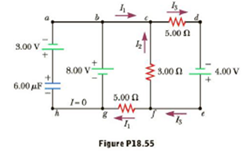Chapter 18, Problem 55AP

Chapter
Section
Textbook Problem

The circuit in Figure P18.55 has been connected for several seconds. Find the current (a) in the 4.00-V battery,(b) in the 3.00-Ω resistor,(c)in the 8.00-V battery, and (d)in the 3.00-V battery.(e)Find the charge on the capacitor.To determine

The value of R.

Explanation

Given info: The power dissipated by the resistance R is 20 W.

The circuit is given as,

• I is the current flowing through the circuit.
• I1 is the current flowing through the resistance R.

Applying Kirchhoff’s loop rule in loop 1,

(5.0Ω)I+(30Ω)(II1)=75V7I6I1=15A

Applying Kirchhoff’s loop rule in loop 2,

(30Ω)(II1)=(40Ω+R)I1I=I1(70Ω+R30Ω)

From the above equations,

I1=450A310+7R

Formula to calculate the power dissipated by the resistance R is,

PR=I12R

Substitute 20 W for PR and re-arrange

Still sussing out bartleby?

Check out a sample textbook solution.

See a sample solution

The Solution to Your Study Problems

Bartleby provides explanations to thousands of textbook problems written by our experts, many with advanced degrees!

Get Started

Taking supplements of fish oil is recommended for those who don't like fish. T F

Nutrition: Concepts and Controversies - Standalone book (MindTap Course List)

Describe diffusion in terms or entropy.

Biology: The Unity and Diversity of Life (MindTap Course List)

What are the general functions of trace minerals in the body?

Chemistry for Today: General, Organic, and Biochemistry